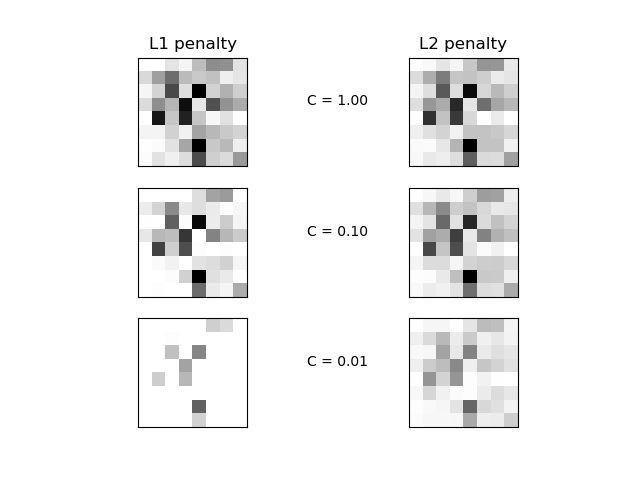# L1 Penalty and Sparsity in Logistic Regression¶

Comparison of the sparsity (percentage of zero coefficients) of solutions when L1 and L2 penalty are used for different values of C. We can see that large values of C give more freedom to the model. Conversely, smaller values of C constrain the model more. In the L1 penalty case, this leads to sparser solutions.

We classify 8x8 images of digits into two classes: 0-4 against 5-9. The visualization shows coefficients of the models for varying C.Out:

C=1.00
Sparsity with L1 penalty: 4.69%
score with L1 penalty: 0.9082
Sparsity with L2 penalty: 4.69%
score with L2 penalty: 0.9048
C=0.10
Sparsity with L1 penalty: 28.12%
score with L1 penalty: 0.9026
Sparsity with L2 penalty: 4.69%
score with L2 penalty: 0.9021
C=0.01
Sparsity with L1 penalty: 84.38%
score with L1 penalty: 0.8625
Sparsity with L2 penalty: 4.69%
score with L2 penalty: 0.8898


print(__doc__)

# Authors: Alexandre Gramfort <alexandre.gramfort@inria.fr>
#          Mathieu Blondel <mathieu@mblondel.org>
#          Andreas Mueller <amueller@ais.uni-bonn.de>

import numpy as np
import matplotlib.pyplot as plt

from sklearn.linear_model import LogisticRegression
from sklearn import datasets
from sklearn.preprocessing import StandardScaler

X, y = digits.data, digits.target
X = StandardScaler().fit_transform(X)

# classify small against large digits
y = (y > 4).astype(np.int)

# Set regularization parameter
for i, C in enumerate((1, 0.1, 0.01)):
# turn down tolerance for short training time
clf_l1_LR = LogisticRegression(C=C, penalty='l1', tol=0.01, solver='saga')
clf_l2_LR = LogisticRegression(C=C, penalty='l2', tol=0.01, solver='saga')
clf_l1_LR.fit(X, y)
clf_l2_LR.fit(X, y)

coef_l1_LR = clf_l1_LR.coef_.ravel()
coef_l2_LR = clf_l2_LR.coef_.ravel()

# coef_l1_LR contains zeros due to the
# L1 sparsity inducing norm

sparsity_l1_LR = np.mean(coef_l1_LR == 0) * 100
sparsity_l2_LR = np.mean(coef_l2_LR == 0) * 100

print("C=%.2f" % C)
print("Sparsity with L1 penalty: %.2f%%" % sparsity_l1_LR)
print("score with L1 penalty: %.4f" % clf_l1_LR.score(X, y))
print("Sparsity with L2 penalty: %.2f%%" % sparsity_l2_LR)
print("score with L2 penalty: %.4f" % clf_l2_LR.score(X, y))

l1_plot = plt.subplot(3, 2, 2 * i + 1)
l2_plot = plt.subplot(3, 2, 2 * (i + 1))
if i == 0:
l1_plot.set_title("L1 penalty")
l2_plot.set_title("L2 penalty")

l1_plot.imshow(np.abs(coef_l1_LR.reshape(8, 8)), interpolation='nearest',
cmap='binary', vmax=1, vmin=0)
l2_plot.imshow(np.abs(coef_l2_LR.reshape(8, 8)), interpolation='nearest',
cmap='binary', vmax=1, vmin=0)
plt.text(-8, 3, "C = %.2f" % C)

l1_plot.set_xticks(())
l1_plot.set_yticks(())
l2_plot.set_xticks(())
l2_plot.set_yticks(())

plt.show()


Total running time of the script: ( 0 minutes 0.781 seconds)

Gallery generated by Sphinx-Gallery